# Applications of Exponential Functions Mr Miehl miehlmtesd net

• Slides: 11Applications of Exponential Functions Mr. Miehl [email protected] netObjectives To solve real-life application problems using the properties of exponents and exponential functions.Why study exponential functions? Exponential functions are used in our real world to measure growth, interest, and decay. Growth obeys exponential functions. Ex: rumors, human population, bacteria, computer technology, nuclear chain reactions, compound interest Decay obeys exponential functions. Ex: Carbon-14 dating, half-life, Newton’s Law of Cooling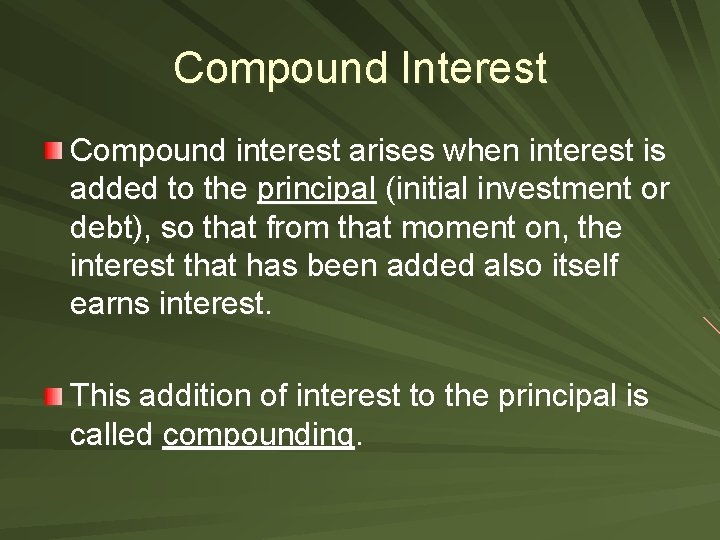Compound Interest Compound interest arises when interest is added to the principal (initial investment or debt), so that from that moment on, the interest that has been added also itself earns interest. This addition of interest to the principal is called compounding.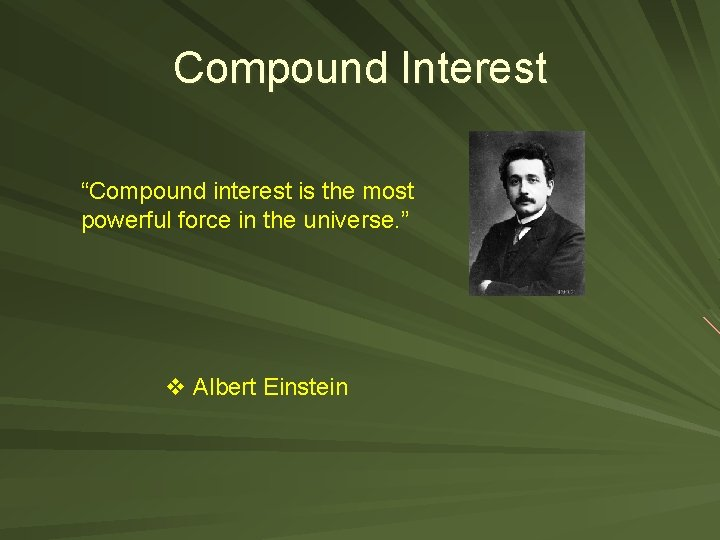Compound Interest “Compound interest is the most powerful force in the universe. ” v Albert Einstein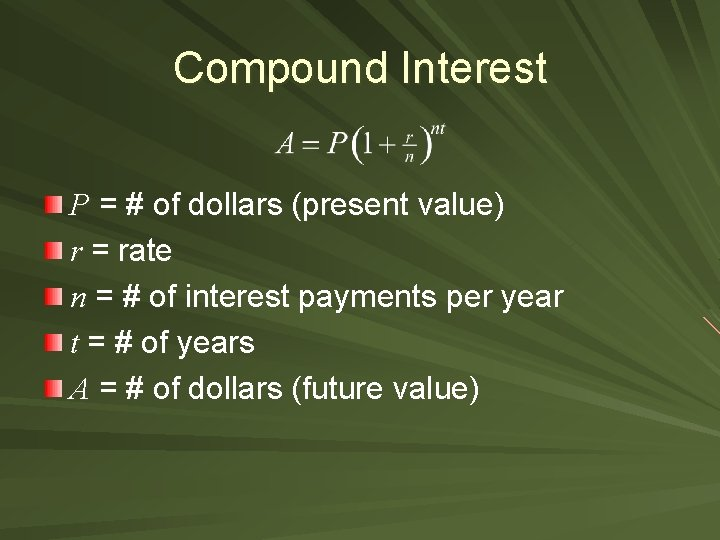Compound Interest P = # of dollars (present value) r = rate n = # of interest payments per year t = # of years A = # of dollars (future value)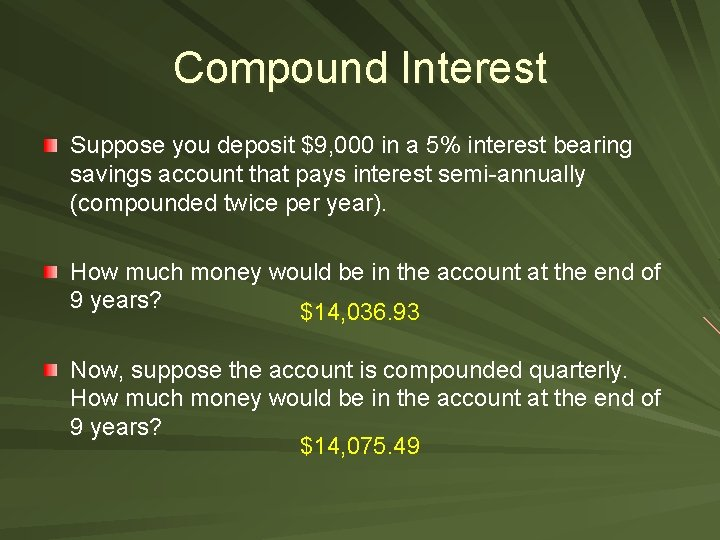Compound Interest Suppose you deposit \$9, 000 in a 5% interest bearing savings account that pays interest semi-annually (compounded twice per year). How much money would be in the account at the end of 9 years? \$14, 036. 93 Now, suppose the account is compounded quarterly. How much money would be in the account at the end of 9 years? \$14, 075. 49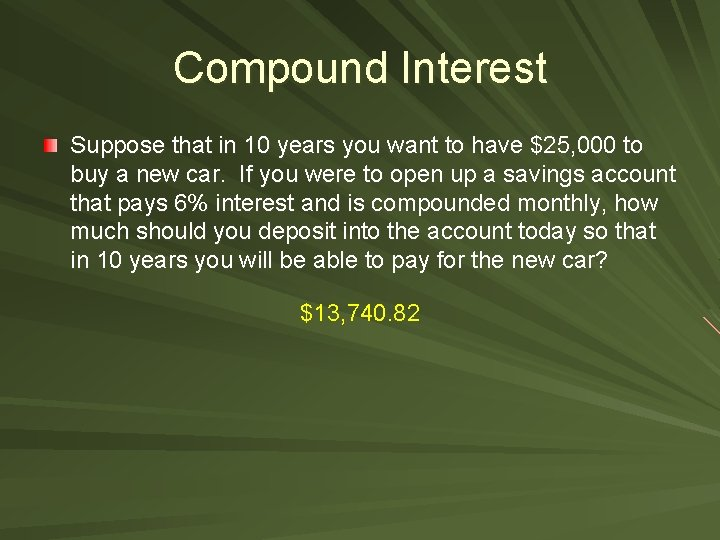Compound Interest Suppose that in 10 years you want to have \$25, 000 to buy a new car. If you were to open up a savings account that pays 6% interest and is compounded monthly, how much should you deposit into the account today so that in 10 years you will be able to pay for the new car? \$13, 740. 82Compound Interest Suppose you want to invest \$100, 000 for 6 months into an account that compounds monthly, and at the end you want to have \$102, 000. What does the account’s interest rate need to be? 4%Continuous Compound Interest P = # of dollars (present value) e = 2. 71828169… r = rate t = # of years A = # of dollars (future value)Continuous Compound Interest What is the total amount for an investment of \$2, 000 invested at 7% for 5 years compounded continuously? \$2, 838. 14